November 24, 2021

# Class 10 Maths Chapter 4 Quadratic Equations All Study Material

In chapter 1 polynomials we learned that based on the degree, an equation can be classified as linear, quadratic, or cubic. In that chapter, we studied some basics of quadratic equations like how they are written and what is their degree, etc. But, in Class 10 Maths Chapter 4 Quadratic Equations we will study these equations in deep and discover different formulas and methods to solve them.

Just to set a rhythm for this chapter, let’s get familiar with a few terms in case if you have not yet started 2nd chapter:

Degree: degree is the highest power of a variable in an equation.
Roots of an equation: The roots of an equation are simply its zeros, meaning the values putting which the whole equation will become zero.

## Class 10 Maths Chapter 4 Quadratic Equations

Before proceeding to explain different methods by which a quadratic equation can be solved, it’s important that we must know about all the technical terms that will be used throughout this chapter. So, in addition to the terms given above, here are a couple of more.

Factorization: Factorization is the process of breaking a complex entity into a product of another entity, or factors. These factors when multiplied together give the original entity. In simple words, breaking an equation into smaller parts is called factorization.

A quadratic equation is simply an algebraic expression or a polynomial equation in which the degree is 2. Please note that equations with degrees more than or less than 2 will not be considered quadratic equations.

the word ‘quad’ means square since the degree in a quadratic equation is 2, hence the name quadratic. The general form of a quadratic equation is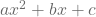, this is also the standard form of a quadratic equation where the variable with degree 2 is written first and then with degree 1, and lastly the constant.

It’s not important that the quadratic equation has to be in standard form, the only thing that matters is that the degree should not be more than 2.

# Solution of a Quadratic Equation by Factorisation

In the method of solving the quadratic equation, we use the factorization method. In this, we find the roots or zeroes of a quadratic equation by factorizing the equation into two linear factors and then equating each factor to zero. This can be done by using the middle term splitting method that we had learned in earlier classes. For example,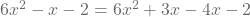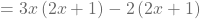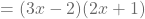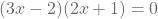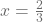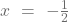### Class 10 Maths Chapter 4 Quadratic Equations Explanation part – 1

There is one more method to solve the quadratic equations i.e by completing the square. In this section we will learn this method in detail.

# Solution of a Quadratic Equation by Completing the Square

We know that square means multiplying a value with itself, or we can say that x times x is the square of x. But explaining how completing the square of a quadratic equation will solve is difficult, therefore, we will use an example and a step-by-step approach to explain this.

Let’s assume that we have to find the roots of the quadratic equation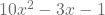.

The key to using this method is to remember that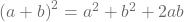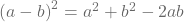Now, we will develop the steps to solve the equation based on these formulas.

Step 1: divide all the terms of the equation with the coefficient of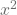. In the above equation, 10 is the coefficient of, therefore, we will divide all the terms of this equation by 10.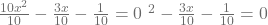Step 2: Now we first multiple the coefficient of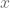with, then add and subtract the square of the result in the equation.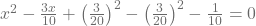Step 3: If you look closely, then you will notice that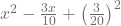makes the formula of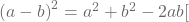. And this is why we can write it as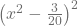. So, now the equation will look something like this: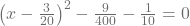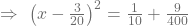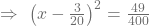Step 4: Now we will put square root to both sides of the equation. Here is what we will get after executing this step in our equation: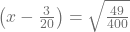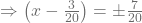Note that we have put both plus and minus signs on the left side of the equation because we know that the square of minus is also plus.

Step 5: Now will solve the obtained equation for both plus and minus to find its roots.

 For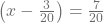For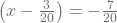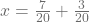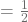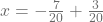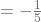### Class 10 Maths Chapter 4 Quadratic Equations Explination – part 2

Based on the completing square method, one of the important formulas that we will learn in class 10 maths chapter 4 quadratic equations is the quadratic formula. This is an important concept that we will use a lot in further classes, which means that you must get it right. Though, it is not an independent topic, but, considering the importance of this topic, we will treat it as a separate topic.

The quadratic formula can be derived from the completing square method that we saw above. There we saw, all the steps involved in finding the roots of a quadratic equation, but, we can skip all those steps if use the quadratic formula.

If we have an equation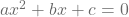, then we can use the following formula to find out the roots of this equation.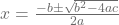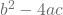is also known as discriminant since it determines if the quadratic equation has real roots or not. In the following points, we will see the nature of roots.

# Nature of roots

Based on the value of the discriminant the nature of the root can be determined in the following ways:

1. if the discriminant is greater than 0, then the equation will have two distinct real roots.
2. If the discriminant is equal to 0, then the equation will have two equal and real roots.
3. And if the discriminant is less than 0 then the equation will have no real roots.

Now let’s see the pattern or blueprint of the class 10 maths chapter 4 quadratic equation.

Maths Chapter 3 Blueprint For class 10 (Pair of linear equations in two variables)
1 Marker 2 question
3 Marker 1 question
Total questions 3
Total marks 5

The total worth of this chapter in terms of the board exam is 5 marks for 3 questions. Out of the 3 questions, there will be two 1 mark questions, and one 3 mark question. Please note that this marking scheme may vary in board exams.